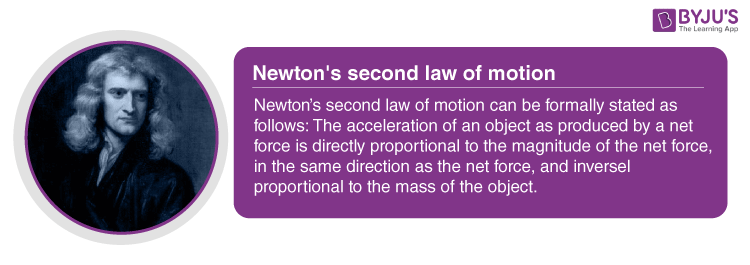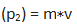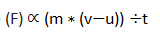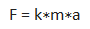# Mathematical Formulation Of Second Law Of Motion

We often observe, that if the same magnitude of the force is used to push two blocks of wood, where one of the blocks is heavier than the other, the rate of change of position of the lighter block will be more than the heavier ones. Similarly, when two same forces are applied to push a car and a bus, the car will have more acceleration compared to the bus. From these examples, it is clear that the acceleration gained by an object when subjected to the same magnitude of the force is a factor of the mass of the object. When a car is given a momentary jerk, it may not move from its initial position whereas when an extended and continuous force of the same magnitude is applied on the car, it experiences a displacement. With this examples, we can conclude that the impact produced by an object depends on its mass and velocity i.e., its momentum and the time rate at which the change in momentum is occurring. The second law of motion is used to validate this phenomenon. In this section, we shall learn about the formulation of the second law of motion.## Newton’s Second Law of Motion

Newton’s second law of motion can be formally stated as follows: The acceleration of an object as produced by a net force is directly proportional to the magnitude of the net force, in the same direction as the net force, and inversely proportional to the mass of the object.

## Second Law of Motion Formula

Let us consider an object of mass m, moving along a straight line with an initial velocity u. Let us say, after a certain time t, with a constant acceleration, the final velocity becomes v. Here we see that, the initial momentumThe final momentumThe change in momentum can be written asAs we know, the rate of change of momentum with respect to time is proportional to the applied force. The applied forceOr,as acceleration (a) = rate of change of velocity with respect to time.Above is the second law of motion formula.

### Notations Used In The Formula

• F is the force
• k is the constant of proportionality
• a is the acceleration

The SI units of mass and acceleration are kg and m.s-2 respectively.
So,The second law of motion gives us a method to measure the force acting on an object as a product of the mass of the object and the acceleration of the object which is the change in velocity with respect to time.